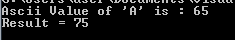Typecasting in C Programming

Typecasting

Suppose we need to add two numbers where one is an integer value (whole number) and other one is a decimal number. Then the result will be a decimal number. But imagine in a program we have declared datatype of result as integer, but actually it has to store floating number. In such case we override the datatype of the result variable by typecasting it to float from its original integer value.

Consider the below program without any typecasting. Here numbers get added even though they are having different datatypes. But when we compile below program, it shows a warning message saying that there would be a loss of data. When we check the result, even though it shows correct result (when %d is used – datatype of intResult), but fails to show decimal values. In second case, when we try to display the data as float, even though result is float, intResult fails to show the correct result. Instead, it shows answer as zero – loss of data. Hence just using different datatype notations while printing the result, will not give correct result. It does not automatically converts its datatype rather there is a loss of data.

//Program without typecasting
#include

void main(){
int intNum1 = 10, intResult =0;
float flNum2 = 22.45;

intResult = intNum1 + flNum2;

printf("Result = %d\n", intResult); // no typecasting of result
printf("Result = %f\n", intResult); // datatype is considered as float here, but not type casted
}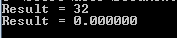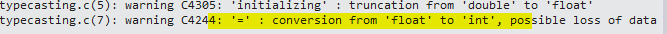In order to overcome above issue, we need to explicitly change the datatype of the variables to get the correct result. Changing the datatype of the variable is valid only for that expression. Once that expression is executed, that variable still will have the original declared datatype. In above example, intResult needs to be type casted to float.

//Program without typecasting
#include

void main(){
int intNum1 = 10;
float flNum2 = 22.45, flResult=0;

flResult =intNum1 + flNum2; // autoamatically type casts the result value now

printf("Result = %f\n", flResult);
}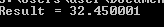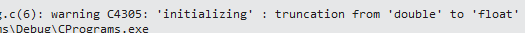Figure 1: No warning message

C Inputs and Outputs in C Programming

In the above case, the datatype of intNum1 is implicilty type casted into float and then both the numbers are added to get floating result. If we need to typecast them to explicitly to float or some other datatype, then we need to explicitly put the datatype that we want before the variable name.

//Program without typecasting
#include

void main(){
int intNum1 = 10;
float flNum2 = 22.45, flResult=0;

flResult =(float)intNum1 + flNum2; // explicitly typecast intNum1 to float

printf("Result = %f\n", flResult);
}

Here also result will be same and no warning message too.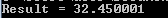We can type cast the variables from lower size value to higher size value. This is called integer promotion. The order of size of the datatype is like below :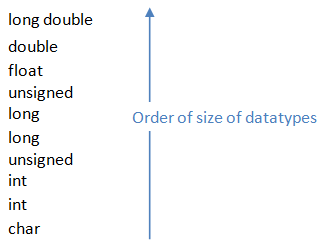Consider another example where we add one number to another character. Here character is of 1 byte and is implicitly converted to integer. That means alphabet is converted to get its ASCII value and is added with the number.

//Program without typecasting
#include

void main(){
int intNum = 10, intResult;
char chrAlpha = 'A';

intResult = intNum + chrAlpha; // implicilty converts chrAlpha into integer

printf("Ascii Value of 'A' is : %d\n", chrAlpha);
printf("Result = %d\n", intResult);
}